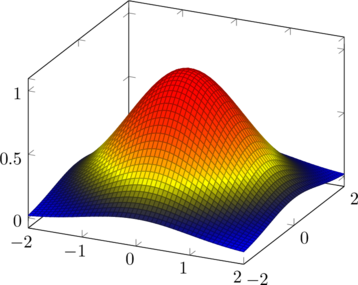LaTeX sample page

We have LaTeX support on this website thanks to the QuickLaTeX plugin. See that link for reference.

• To write inline formulas in the text, simply enclose them between dollar signs.
$x^2+y^2=z^2$

is displayed as.

• To write centered display-formulas, use double dollar signs.
 $$\int_{\D \Omega} \omega = \int_{\Omega}d\omega$$

is rendered as:• To write commutative diagrams, use tikzcd. For example,
 \begin{tikzcd}
E \ar{r}{f'} \ar{d}{p} & F\ar{d}{q} \\
A \arrow[r, bend left=40, ""{name=U, below}] \arrow[r, bend right=40, ""{name=D}] & B
\arrow[Rightarrow, from=U, to=D]
\end{tikzcd}

is rendered as:• To draw graphs, use tikzpicture/pgfplots (manual here). For example, this code:
 \begin{tikzpicture}
\begin{axis}
\end{axis}
\end{tikzpicture}

is rendered as:• It’s important not to end the line twice in math mode. Either use the “text” visualization (as opposed to “visual”, top right corner of the editing page), or hold Shift while pressing Enter.
• If the equations look cut on your mobile screen, tap on them and slide left. You will be able to see everything!
• If you want to add a preamble, do it in the beginning of the page, as you would do in a LaTeX document. The following packages are already automatically loaded: amsfonts, amsmath, amssymb, amsthm, bbm, latexsym, mathtools, pgfplots, tikz, tikzcd, verbatim.

Try it out in the sandbox!

•Sidebar